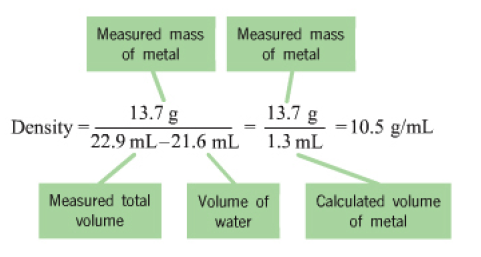# Problem: Part B. You add 6.6 g iron to 24.00 mL of water and observe that the volume of iron and water together is 24.84 mL. Calculate the density of iron.Express your answer to two significant figures with the appropriate unitsThe density of a substance is an intrinsic property and is independent of the amount of substance present. It is often used to identify a substance.Water displacement can be used to find the volume of a solid by placing the object in a known volume of water. When the object is added to water, the measured total volume increases. This final volume is equal to the volume of the initial water plus the volume of the solid object added. To determine the volume of the water that is displaced by the object subtract the initial volume from the final volume. Using a scale to measure the mass of the object, you can now calculate the density of the object as its mass divided by its volume.For example, if you add 13.7 g of silver to 20.0 mL of water, the final volume observed in the measuring cylinder is 21.3 mL. The change in volume is 21.3 mL − 20.0 mL = 1.3 mL. The density of silver is thus13.71.3 mL=10.5 gmLEach calculation is shown after clicking to calculate the density in the simulation.

###### FREE Expert Solution
86% (124 ratings)View Complete Written Solution
###### Problem Details

Part B. You add 6.6 g iron to 24.00 mL of water and observe that the volume of iron and water together is 24.84 mL. Calculate the density of iron.

The density of a substance is an intrinsic property and is independent of the amount of substance present. It is often used to identify a substance.

Water displacement can be used to find the volume of a solid by placing the object in a known volume of water. When the object is added to water, the measured total volume increases. This final volume is equal to the volume of the initial water plus the volume of the solid object added. To determine the volume of the water that is displaced by the object subtract the initial volume from the final volume. Using a scale to measure the mass of the object, you can now calculate the density of the object as its mass divided by its volume.

For example, if you add 13.7 g of silver to 20.0 mL of water, the final volume observed in the measuring cylinder is 21.3 mL. The change in volume is 21.3 mL − 20.0 mL = 1.3 mL. The density of silver is thus

Each calculation is shown after clicking to calculate the density in the simulation.